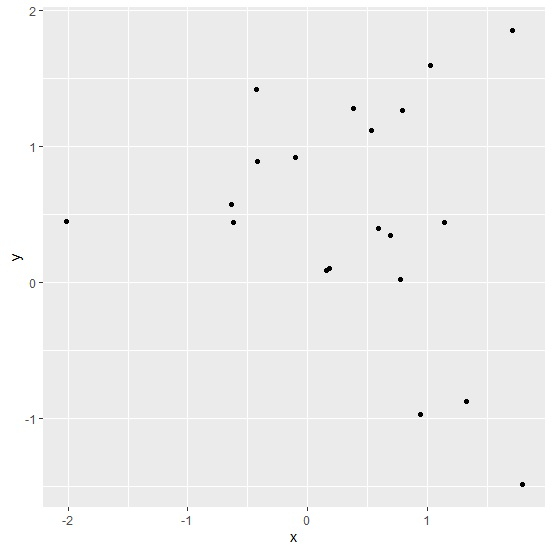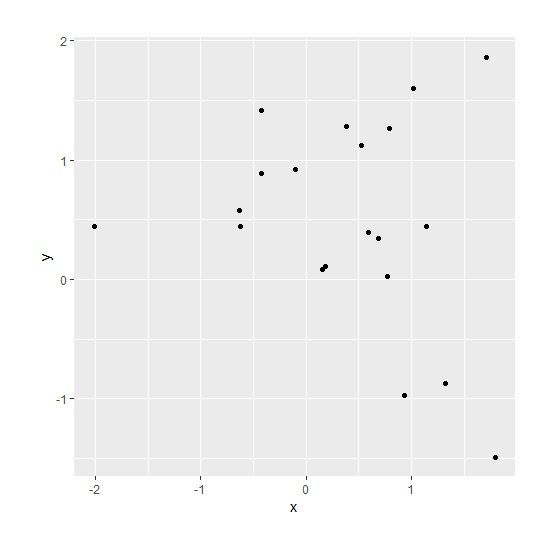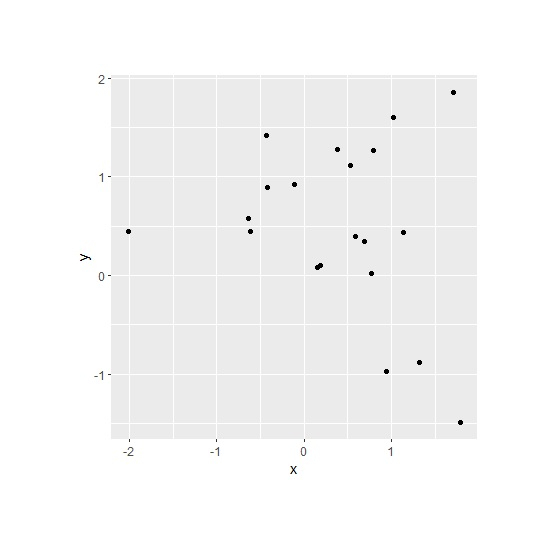# How to change plot area margins using ggplot2 in R?

While creating plots using ggplot2, the plot area is of square shape but we can change our plot area by setting plot.margin in theme function. This is helpful when we want to decrease the plot area and also when the data points are less.

## Example

Consider the below data frame −

> set.seed(1)
> x<-rnorm(20,0.2)
> y<-rnorm(20,0.5)
> df<-data.frame(x,y)

> library(ggplot2)

Creating the scatterplot without changing the plot area margins −

> ggplot(df,aes(x,y))+
+ geom_point()> ggplot(df,aes(x,y))+
+ geom_point()+
+ theme(plot.margin = unit(c(1,1,1,1), "cm"))> ggplot(df,aes(x,y))+
+ geom_point()+
+ theme(plot.margin = unit(c(2,2,2,2), "cm"))